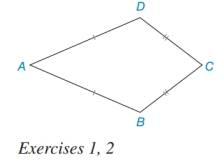Chapter 7.3, Problem 2EElementary Geometry For College St...

7th Edition
Alexander + 2 others
ISBN: 9781337614085

Solutions

Chapter
SectionElementary Geometry For College St...

7th Edition
Alexander + 2 others
ISBN: 9781337614085
Textbook Problem

What condition must be satisfied for it to be possible to circumscribe a circle about kite ABCD?To determine

To find:

The condition must be satisfied for it to be possible to circumscribe a circle about kite ABCD.

Explanation

The circle can be circumscribed about kite ABCD if and only if the perpendicular bisectors of AB, BC, CD and DA meet at one point. The point where the bisectors meet is called the circumcenter...

Still sussing out bartleby?

Check out a sample textbook solution.

See a sample solution

The Solution to Your Study Problems

Bartleby provides explanations to thousands of textbook problems written by our experts, many with advanced degrees!

Get Started

Change each percent to a decimal: 15%

Elementary Technical Mathematics

Evaluate the integrals in Problems 1-26.

Mathematical Applications for the Management, Life, and Social Sciences

Expand each expression in Exercises 122. (y+3)(y+4)

Finite Mathematics and Applied Calculus (MindTap Course List)

Simplify each power of i. i22

Trigonometry (MindTap Course List)

True or False: n=21(lnn)n converges.

Study Guide for Stewart's Single Variable Calculus: Early Transcendentals, 8th